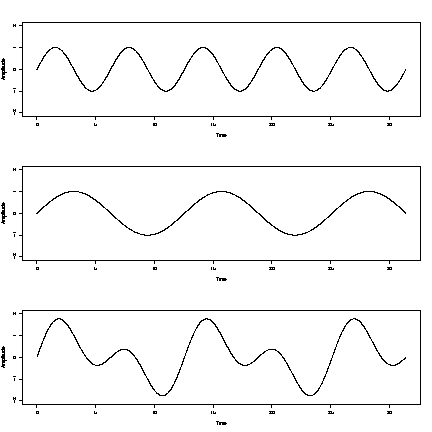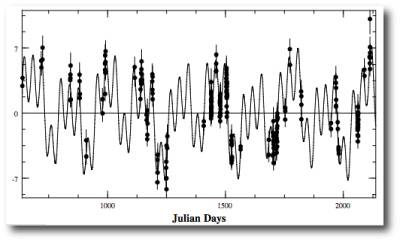# Detecting more than 1 exoplanet using Radial Velocity Method.

avito009
How do we detect if there is more than 1 exoplanet orbiting a star using radial velocity method?

I read this but I didnt understand it. Can someone explain the answer in Laymans terms?

"Multiple planets will cause a stars radial velocity curve to show two periods: a long gradual change due to the outer planet, and a shorter, sharper change due to the inner planet. The two periods are superimposed on each other, giving the radial velocity curve a complex shape".

## Answers and Replies

Imagine there's just a single planet in a circular orbit. It and the star both orbit their mutual centre of mass, and the motion of the star causes variations in detected light wavelengths due to Doppler shift. This lets you calculate the radial velocity.
If you draw a graph of the star velocity against time, in the case above you'd get a sinusoid with the period reflecting that of the planet's orbital period.

Now, imagine another planet, with a different orbital period. If, again, it were the only planet, it'd generate another sinusoid, but with a different period(and depending on mass - amplitude).

With both the planets present, you'd have a velocity graph that represents both of the graphs superimposed, like so:As with any other function addition, you just take the value(V) of the first one at any given time, and add it to the value of the second one at that time, and plot the result on a new graph.

The more planets you add, the more complex the resulting function becomes. This one is for a simulated system resembling that of Jupiter and its Galilean moons:Elliptical orbits produce a somewhat different velocity graph than a simple sine function, but the principle is the same.

How the detection process works, is you draw the function* from observations, and then try to construct such a model of the system that would result in matching the graph. You try to figure out how many, how large, and how distant planets you need to add for all of their contributions to add up to what you see.

*that's not entirely accurate, but let's not get into details

Last edited:
snorkack
Note that if the periods are in 1:2 resonance, the resulting orbit is hard to distinguish from a single planet on an elliptical orbit.